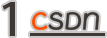### Knowledge Graph

• 「精确性」：为物品 item 引入了更多的语义关系，可以深层次地发现用户兴趣

• 「多样性」：提供了不同的关系连接种类，有利于推荐结果的发散，避免推荐结果局限于单一类型

• 「可解释性」：连接用户的历史记录和推荐结果，从而提高用户对推荐结果的满意度和接受度，增强用户对推荐系统的信任。

embedding 方法主要可以分为 translational distance 方法和 semantic matching 方法两种，前者是学习从头实体到尾实体的空间关系变换（如 TransE 等系列），后者则是直接用神经网络对语义相似度进行计算

• 「图简化」 如何处理 KG 带来的多种实体和关系，按需要简化虽然可能会损失部分信息但对效率是必要的，如只对 user-user 或者 item-item 关系简化子图。

• 「多关系传播」KG 的特点就是多关系，不过现有可以用 attention 来区分不同关系的重要性，为邻居加权。

• 「用户整合」将角色引入图结构，由于 KG 是外部信号，但是否也可以将用户也融入为一种实体变成内在产物呢？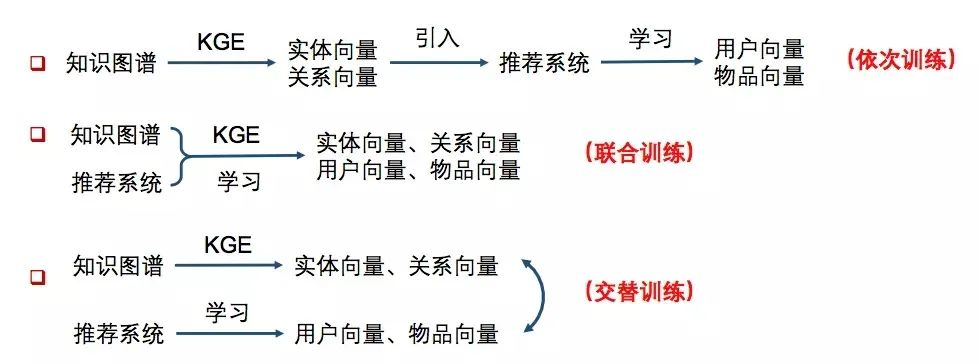• 依次学习：使用知识图谱特征学习得到实体向量和关系向量，然后将这些低维向量（TransR方法等），引入推荐系统再做后面的处理。即只把知识图谱作为一个 side info，多一维特征的处理方式。

• 联合学习：将知识图谱特征学习和推荐算法的目标函数结合，使用端到端（end-to-end）的方法进行联合学习。即把知识图谱的损失也纳入到最后的损失函数联合训练。

• 交替学习：将知识图谱特征学习和推荐算法视为两个分离但又相关的任务，使用多任务学习（multi-task learning）的框架进行交替学习。这样可以让 KG 和 RC 在某种程度上融合的更加深入。

• TransE：即使其满足 h + r ≈ t，尾实体是头实体通过关系平移(翻译)得到的，但它不适合多对一和多对多，所以导致 TransE 在复杂关系上的表现差。公式如下：

• TransH 模型：即将实体投影到由关系构成的超平面上。值得注意的是它是非对称映射

• TransR 模型：该模型则认为实体和关系存在语义差异，它们应该在不同的语义空间。此外，不同的关系应该构成不同的语义空间，因此 TransR 通过关系投影矩阵，将实体空间转换到相应的关系空间。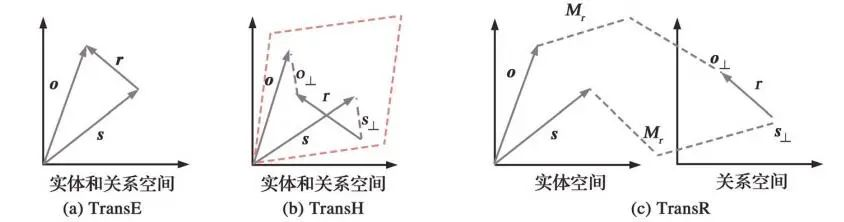• TransD 模型：该模型认为头尾实体的属性通常有比较大的差异，因此它们应该拥有不同的关系投影矩阵。此外还考虑矩阵运算比较耗时，TransD 将矩阵乘法改成了向量乘法，从而提升了运算速度。

• NTN 模型：将每一个实体用其实体名称的词向量平均值来表示，可以共享相似实体名称中的文本信息。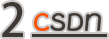### CKE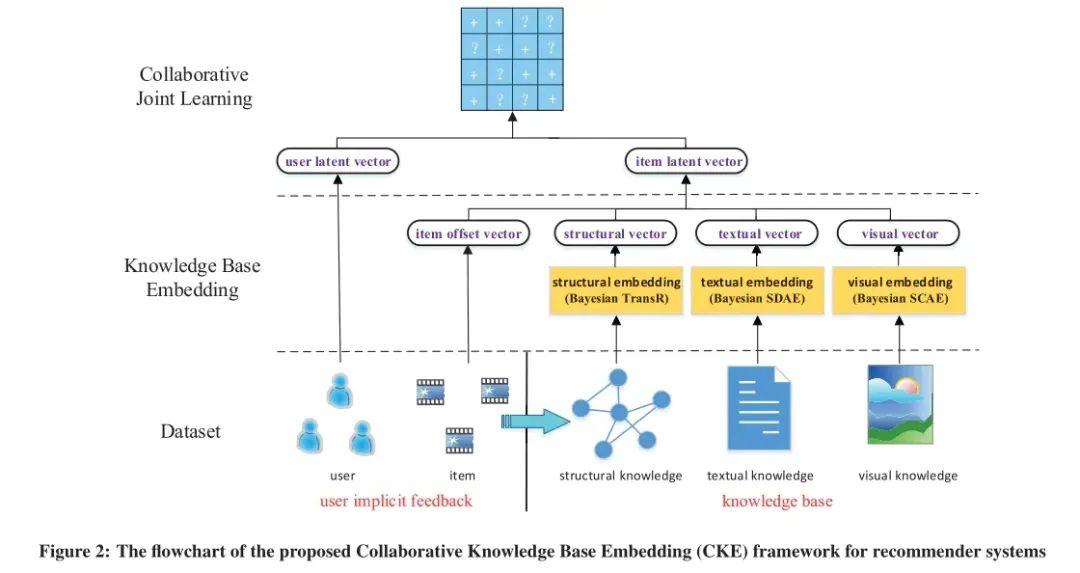##### 视觉知识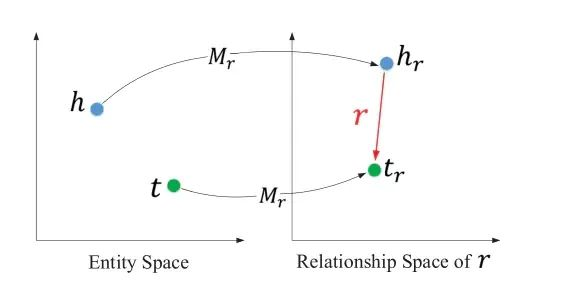``````#TransR
def projection_transR_pytorch(original, proj_matrix):
ent_embedding_size = original.shape
rel_embedding_size = proj_matrix.shape // ent_embedding_size
original = original.view(-1, ent_embedding_size, 1)
#借助一个投影矩阵就行
proj_matrix = proj_matrix.view(-1, rel_embedding_size, ent_embedding_size)
``````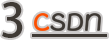### RippleNet

Ripple Network 模拟了用户兴趣在知识图谱上的传播过程，整个过程类似于水波的传播，如上图从实体 Forrest Gump 开始一跳 hop1，二跳 hop2 做传播，同时权重递减。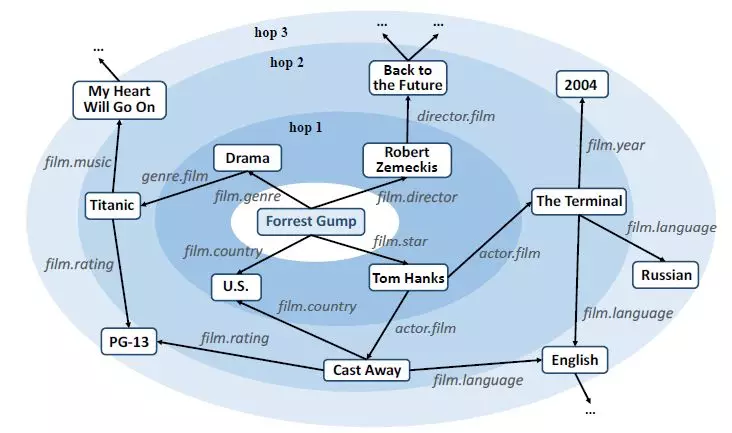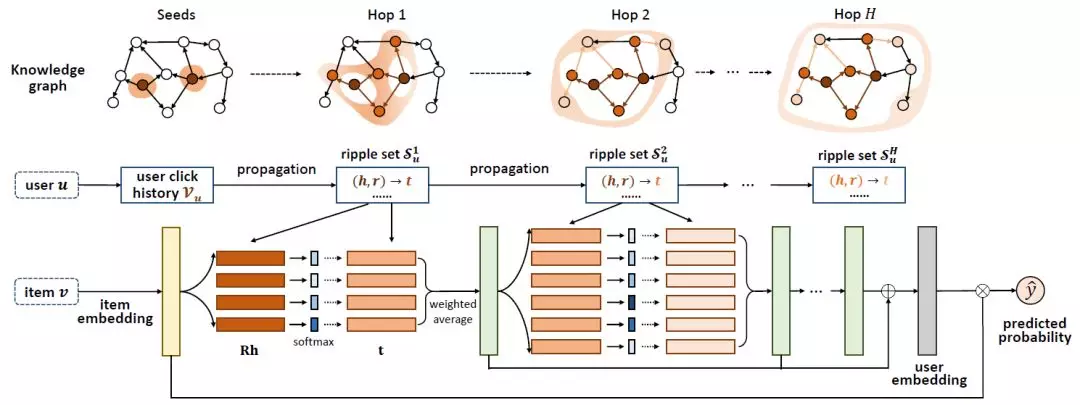v 是物品向量，r 是关系，h 是头节点，三者计算相似度（得到了图片中 Rh 后面的绿色方格）。然后用这个权重对该实体中的尾节点 t 加权就得到了第一跳/扩散的结果：

``````class RippleNet(object):    def __init__(self, args, n_entity, n_relation):        self._parse_args(args, n_entity, n_relation)        self._build_inputs()        self._build_embeddings()        self._build_model()        self._build_loss()        self._build_train()
def _parse_args(self, args, n_entity, n_relation):        self.n_entity = n_entity        self.n_relation = n_relation        self.dim = args.dim        self.n_hop = args.n_hop        self.kge_weight = args.kge_weight        self.l2_weight = args.l2_weight        self.lr = args.lr        self.n_memory = args.n_memory        self.item_update_mode = args.item_update_mode        self.using_all_hops = args.using_all_hops
def _build_inputs(self):        #输入有items id，labels和用户每一跳的ripple set记录        self.items = tf.placeholder(dtype=tf.int32, shape=[None], name="items")        self.labels = tf.placeholder(dtype=tf.float64, shape=[None], name="labels")        self.memories_h = []        self.memories_r = []        self.memories_t = []
for hop in range(self.n_hop):#每一跳的结果            self.memories_h.append(                tf.placeholder(dtype=tf.int32, shape=[None, self.n_memory], name="memories_h_" + str(hop)))            self.memories_r.append(                tf.placeholder(dtype=tf.int32, shape=[None, self.n_memory], name="memories_r_" + str(hop)))            self.memories_t.append(                tf.placeholder(dtype=tf.int32, shape=[None, self.n_memory], name="memories_t_" + str(hop)))
def _build_embeddings(self):#得到嵌入        self.entity_emb_matrix = tf.get_variable(name="entity_emb_matrix", dtype=tf.float64,                                                 shape=[self.n_entity, self.dim],                                                 initializer=tf.contrib.layers.xavier_initializer())        #relation连接head和tail所以维度是self.dim*self.dim        self.relation_emb_matrix = tf.get_variable(name="relation_emb_matrix", dtype=tf.float64,                                                   shape=[self.n_relation, self.dim, self.dim],                                                   initializer=tf.contrib.layers.xavier_initializer())
def _build_model(self):        # transformation matrix for updating item embeddings at the end of each hop        # 更新item嵌入的转换矩阵，这个不一定是必要的，可以使用直接替换或者加和策略。        self.transform_matrix = tf.get_variable(name="transform_matrix", shape=[self.dim, self.dim], dtype=tf.float64,                                                initializer=tf.contrib.layers.xavier_initializer())
# [batch size, dim]，得到item的嵌入        self.item_embeddings = tf.nn.embedding_lookup(self.entity_emb_matrix, self.items)
self.h_emb_list = []        self.r_emb_list = []        self.t_emb_list = []        for i in range(self.n_hop):#得到每一跳的实体，关系嵌入list            # [batch size, n_memory, dim]            self.h_emb_list.append(tf.nn.embedding_lookup(self.entity_emb_matrix, self.memories_h[i]))
# [batch size, n_memory, dim, dim]            self.r_emb_list.append(tf.nn.embedding_lookup(self.relation_emb_matrix, self.memories_r[i]))
# [batch size, n_memory, dim]            self.t_emb_list.append(tf.nn.embedding_lookup(self.entity_emb_matrix, self.memories_t[i]))
#得到分数        self.scores = tf.squeeze(self.predict(self.item_embeddings, o_list))        self.scores_normalized = tf.sigmoid(self.scores)
def _key_addressing(self):#得到olist        o_list = []        for hop in range(self.n_hop):#依次计算每一跳            # [batch_size, n_memory, dim, 1]            h_expanded = tf.expand_dims(self.h_emb_list[hop], axis=3)
# [batch_size, n_memory, dim]，计算Rh，使用matmul函数            Rh = tf.squeeze(tf.matmul(self.r_emb_list[hop], h_expanded), axis=3)
# [batch_size, dim, 1]            v = tf.expand_dims(self.item_embeddings, axis=2)
# [batch_size, n_memory]，然后和v内积计算相似度            probs = tf.squeeze(tf.matmul(Rh, v), axis=2)
# [batch_size, n_memory]，softmax输出分数            probs_normalized = tf.nn.softmax(probs)
# [batch_size, n_memory, 1]            probs_expanded = tf.expand_dims(probs_normalized, axis=2)
# [batch_size, dim]，然后分配分数给尾节点得到o            o = tf.reduce_sum(self.t_emb_list[hop] * probs_expanded, axis=1)
#更新Embedding表，并且存好o            self.item_embeddings = self.update_item_embedding(self.item_embeddings, o)            o_list.append(o)        return o_list
def update_item_embedding(self, item_embeddings, o):        #计算完hop之后，更新item的Embedding操作，可以有多种策略        if self.item_update_mode == "replace":#直接换            item_embeddings = o        elif self.item_update_mode == "plus":#加到一起            item_embeddings = item_embeddings + o        elif self.item_update_mode == "replace_transform":#用前面的转换矩阵            item_embeddings = tf.matmul(o, self.transform_matrix)        elif self.item_update_mode == "plus_transform":#用矩阵而且再加到一起            item_embeddings = tf.matmul(item_embeddings + o, self.transform_matrix)        else:            raise Exception("Unknown item updating mode: " + self.item_update_mode)        return item_embeddings
def predict(self, item_embeddings, o_list):        y = o_list[-1]#1只用olist的最后一个向量        if self.using_all_hops:#2或者使用所有向量的相加来代表user            for i in range(self.n_hop - 1):                y += o_list[i]
# [batch_size]，user和item算内积得到预测值        scores = tf.reduce_sum(item_embeddings * y, axis=1)        return scores
def _build_loss(self):#损失函数有三部分        #1用于推荐的对数损失函数        self.base_loss = tf.reduce_mean(tf.nn.sigmoid_cross_entropy_with_logits(labels=self.labels, logits=self.scores))
#2知识图谱表示的损失函数        self.kge_loss = 0        for hop in range(self.n_hop):            h_expanded = tf.expand_dims(self.h_emb_list[hop], axis=2)            t_expanded = tf.expand_dims(self.t_emb_list[hop], axis=3)            hRt = tf.squeeze(tf.matmul(tf.matmul(h_expanded, self.r_emb_list[hop]), t_expanded))            self.kge_loss += tf.reduce_mean(tf.sigmoid(hRt))#为hRt的表示是否得当        self.kge_loss = -self.kge_weight * self.kge_loss
#3正则化损失        self.l2_loss = 0        for hop in range(self.n_hop):            self.l2_loss += tf.reduce_mean(tf.reduce_sum(self.h_emb_list[hop] * self.h_emb_list[hop]))            self.l2_loss += tf.reduce_mean(tf.reduce_sum(self.t_emb_list[hop] * self.t_emb_list[hop]))            self.l2_loss += tf.reduce_mean(tf.reduce_sum(self.r_emb_list[hop] * self.r_emb_list[hop]))            if self.item_update_mode == "replace nonlinear" or self.item_update_mode == "plus nonlinear":                self.l2_loss += tf.nn.l2_loss(self.transform_matrix)        self.l2_loss = self.l2_weight * self.l2_loss
self.loss = self.base_loss + self.kge_loss + self.l2_loss #三者相加
def train(self, sess, feed_dict):#开始训练        return sess.run([self.optimizer, self.loss], feed_dict)
def eval(self, sess, feed_dict):#开始测试        labels, scores = sess.run([self.labels, self.scores_normalized], feed_dict)        #计算auc和acc        auc = roc_auc_score(y_true=labels, y_score=scores)        predictions = [1 if i >= 0.5 else 0 for i in scores]        acc = np.mean(np.equal(predictions, labels))        return auc, acc
``````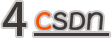### 关于多跳的实现

``````#ripple多跳时，每跳的结果集def get_ripple_set(args, kg, user_history_dict):    print('constructing ripple set ...')
# user -> [(hop_0_heads, hop_0_relations, hop_0_tails), (hop_1_heads, hop_1_relations, hop_1_tails), ...]    ripple_set = collections.defaultdict(list)
for user in user_history_dict:#对于每个用户        for h in range(args.n_hop):#该用户的兴趣在KG多跳hop中            memories_h = []            memories_r = []            memories_t = []
if h == 0:#如果不传播，上一跳的结果就直接是该用户的历史记录                tails_of_last_hop = user_history_dict[user]            else:#去除上一跳的记录                tails_of_last_hop = ripple_set[user][-1]
#去除上一跳的三元组特征            for entity in tails_of_last_hop:                for tail_and_relation in kg[entity]:                    memories_h.append(entity)                    memories_r.append(tail_and_relation)                    memories_t.append(tail_and_relation)
# if the current ripple set of the given user is empty, we simply copy the ripple set of the last hop here            # this won't happen for h = 0, because only the items that appear in the KG have been selected            # this only happens on 154 users in Book-Crossing dataset (since both BX dataset and the KG are sparse)            if len(memories_h) == 0:                ripple_set[user].append(ripple_set[user][-1])            else:                #为每个用户采样固定大小的邻居                replace = len(memories_h) < args.n_memory                indices = np.random.choice(len(memories_h), size=args.n_memory, replace=replace)                memories_h = [memories_h[i] for i in indices]                memories_r = [memories_r[i] for i in indices]                memories_t = [memories_t[i] for i in indices]                ripple_set[user].append((memories_h, memories_r, memories_t))
return ripple_set
``````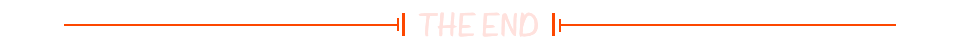``````

☞百度推出开发者搜索 Beta；雷军手机使用时长曝光；苹果败诉，电脑上可以模拟 iOS 系统 | 极客头条☞我们差点就用不上 Java 了！
☞印度永久封禁了微信、百度、TikTok 等 59 款中国 App……

``````20年前，《新程序员》创刊时，我们的心愿是全面关注程序员成长，中国将拥有新一代世界级的程序员。20年后的今天，我们有了新的使命：助力中国IT技术人成长，成就一亿技术人！